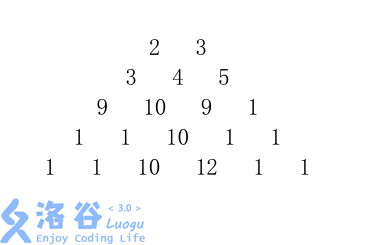P4013 数字梯形问题

• 496通过
• 1.3K提交
• 题目提供者 zzlzk
• 评测方式 云端评测
• 标签 SPFA 图的建立,建图 最大流 网络流24题 O2优化
• 难度 省选/NOI-
• 时空限制 1000ms / 256MB
• 提示：收藏到任务计划后，可在首页查看。

题目描述

给定一个由 $n$ 行数字组成的数字梯形如下图所示。梯形的第一行有 $m$ 个数字。从梯形的顶部的 $m$ 个数字开始，在每个数字处可以沿左下或右下方向移动，形成一条从梯形的顶至底的路径。

分别遵守以下规则：

1. 从梯形的顶至底的 $m$ 条路径互不相交；

2. 从梯形的顶至底的 $m$ 条路径仅在数字结点处相交；

3. 从梯形的顶至底的 $m$ 条路径允许在数字结点相交或边相交。

输入输出格式

输入格式：

第 $1$ 行中有 $2$ 个正整数 $m$ 和 $n$，分别表示数字梯形的第一行有 $m$ 个数字，共有 $n$ 行。接下来的 $n$ 行是数字梯形中各行的数字。

第 $1$ 行有 $m$ 个数字，第 $2$ 行有 $m+1$ 个数字，以此类推。

输出格式：

将按照规则 $1$，规则 $2$，和规则 $3$ 计算出的最大数字总和并输出，每行一个最大总和。

输入输出样例

输入样例#1： 复制
2 5
2 3
3 4 5
9 10 9 1
1 1 10 1 1
1 1 10 12 1 1
输出样例#1： 复制
66
75
77

说明

$1\leq m,n \leq 20$

提示
标程仅供做题后或实在无思路时参考。
请自觉、自律地使用该功能并请对自己的学习负责。
如果发现恶意抄袭标程，将按照I类违反进行处理。